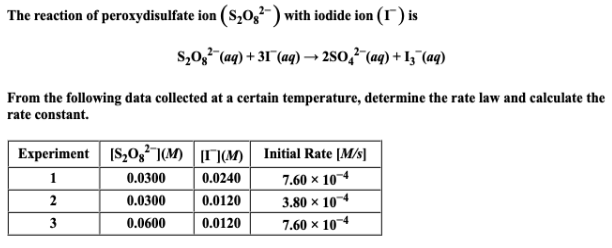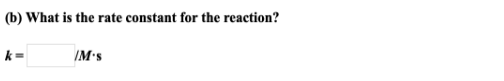# The reaction of peroxydisulfate ion (S2O8^2-) with iodide ion is S2O8^2-(aq) → 2SO4^2-(aq) + I3^-(aq) From the following data collected at a certain temperature, determine the rate law and calculate the rate constant. b) What is the rate constant for the reaction?